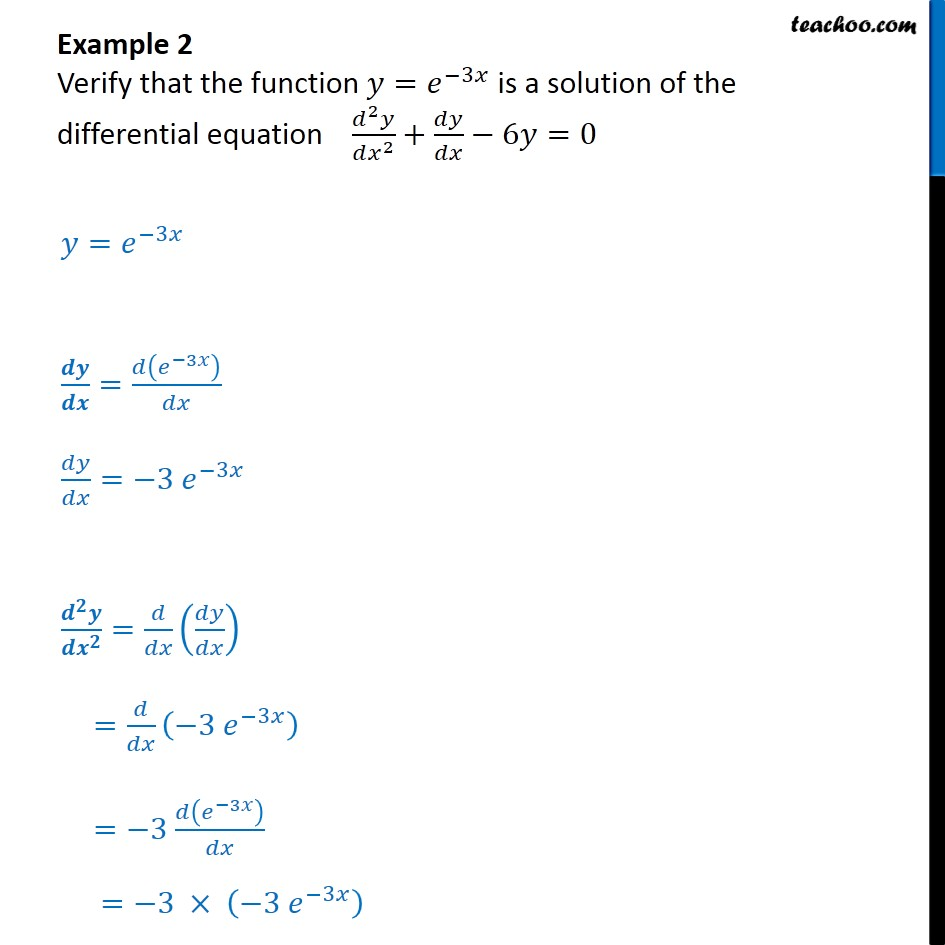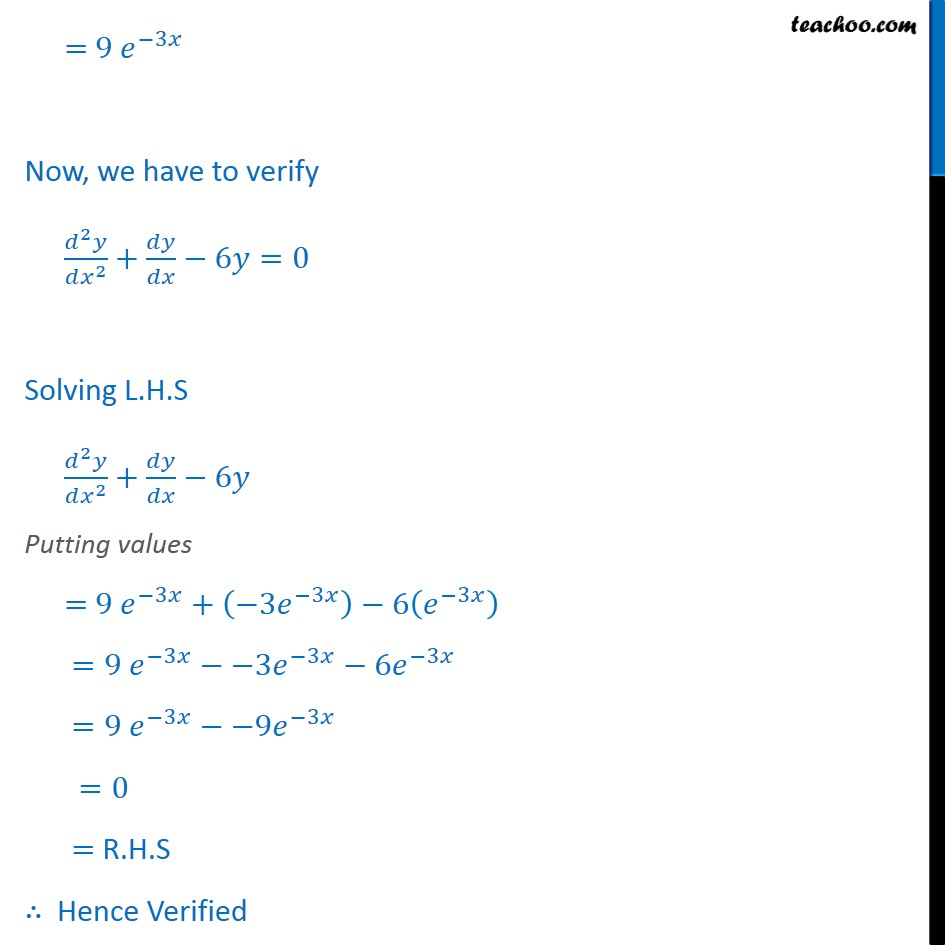1. Chapter 9 Class 12 Differential Equations
2. Serial order wise
3. Examples

Transcript

Example 2 Verify that the function 𝑦= 𝑒﷮−3𝑥﷯ is a solution of the differential equation 𝑑﷮2﷯𝑦﷮𝑑 𝑥﷮2﷯﷯+ 𝑑𝑦﷮𝑑𝑥﷯−6𝑦=0 𝑦= 𝑒﷮−3𝑥﷯ 𝒅𝒚﷮𝒅𝒙﷯= 𝑑 𝑒﷮−3𝑥﷯﷯﷮𝑑𝑥﷯ 𝑑𝑦﷮𝑑𝑥﷯= −3 𝑒﷮−3𝑥﷯ 𝒅﷮𝟐﷯𝒚﷮𝒅 𝒙﷮𝟐﷯﷯= 𝑑﷮𝑑𝑥﷯ 𝑑𝑦﷮𝑑𝑥﷯﷯ = 𝑑﷮𝑑𝑥﷯ −3 𝑒﷮−3𝑥﷯﷯ =−3 𝑑 𝑒﷮−3𝑥﷯﷯﷮𝑑𝑥﷯ =−3 × −3 𝑒﷮−3𝑥﷯﷯ = 9 𝑒﷮−3𝑥﷯ Now, we have to verify 𝑑﷮2﷯𝑦﷮𝑑 𝑥﷮2﷯﷯+ 𝑑𝑦﷮𝑑𝑥﷯−6𝑦=0 Solving L.H.S 𝑑﷮2﷯𝑦﷮𝑑 𝑥﷮2﷯﷯+ 𝑑𝑦﷮𝑑𝑥﷯−6𝑦 Putting values = 9 𝑒﷮−3𝑥﷯+ −3 𝑒﷮−3𝑥﷯﷯−6 𝑒﷮−3𝑥﷯﷯ = 9 𝑒﷮−3𝑥﷯−−3 𝑒﷮−3𝑥﷯−6 𝑒﷮−3𝑥﷯ = 9 𝑒﷮−3𝑥﷯−−9 𝑒﷮−3𝑥﷯ =0 = R.H.S ∴ Hence Verified

Examples